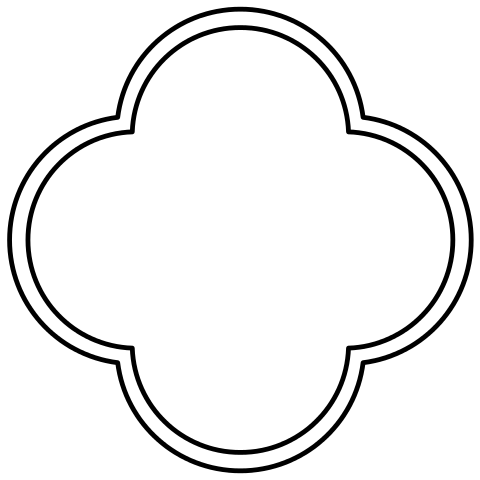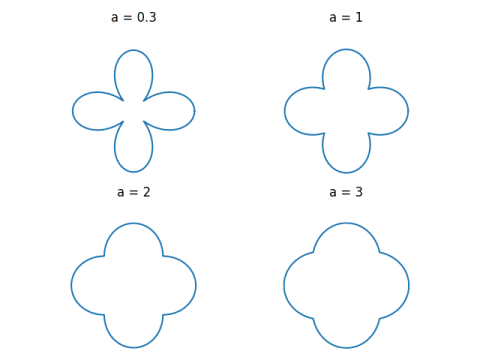# Quatrefoils

I was reading The 99% Invisible City this evening, and there was a section on quatrefoils. Here’s an example of a quatrefoil from Wikipedia.There’s no single shape known as a quatrefoil. It’s a family of shapes that look something like the figure above.

I wondered how you might write a fairly simple mathematical equation to draw a quatrefoil. Some quatrefoils are just squares with semicircles glued on their edges. That’s no fun.

Here’s a polar equation I came up with that looks like a quatrefoil, if you ignore the interior lines.

This is the plot of r = cos(2θ/3).Update: Based on a suggestion in the comments, I’ve written another post on quatrefoils using an equation that has a parameter to control the shape.## 3 thoughts on “Quatrefoils”

1. Paul Clapham

Looks like an interesting book, I put a hold on it at my local public library.

(But don’t expect me to post back with a review, because I’m #22 in the queue for 2 copies which the library doesn’t have yet. And it’s running at less than half speed in these COVID days.)

2. Jan Van lent

Two more ideas.
Contours of |x^2+y^2|-a*(x^2+y^2) give quatrefoils made of elliptical arcs.
A polar plot of a+|cos(2*t)| also produces a quatrefoil, e.g, with a=1 or a=2.

3. Jan Van lent

Another one: contours of
(|x|+|y|+||x|-|y||)/(x^2+y^2).
Based on the conformal mapping 1/z applied to squares.